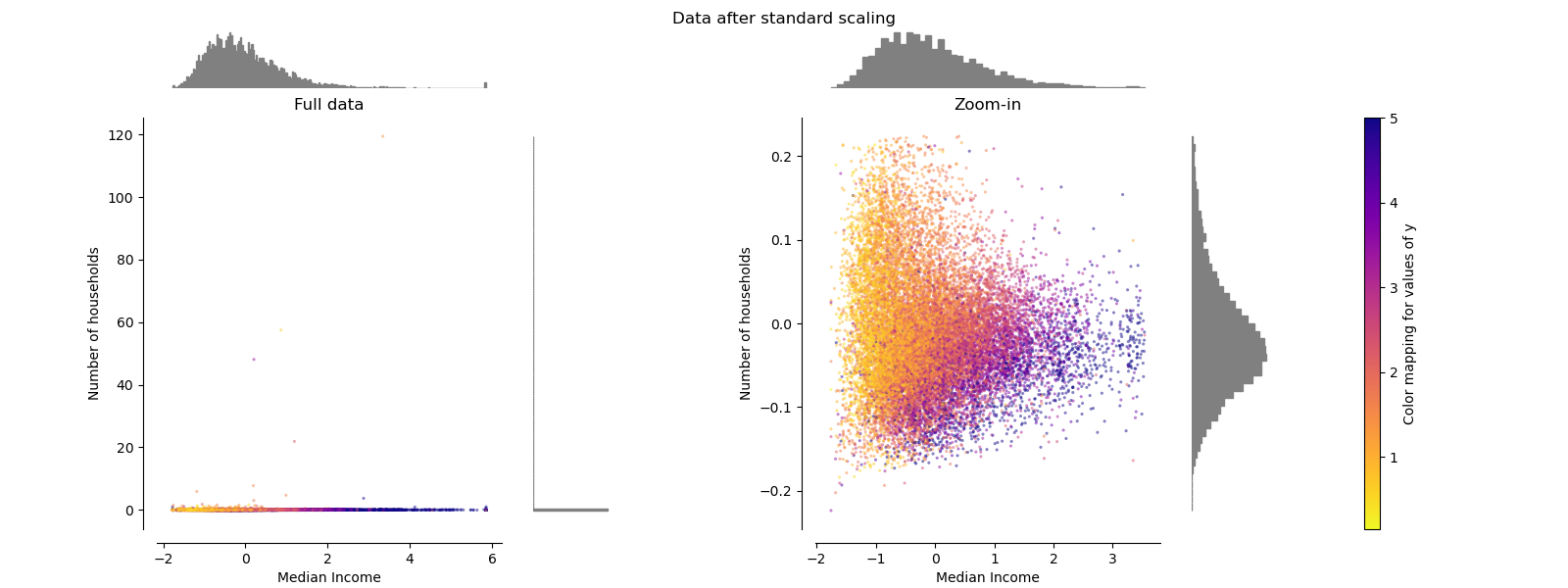sklearn.preprocessing.Normalizer¶

class sklearn.preprocessing.Normalizer(norm='l2', *, copy=True)

norm 用于规范化每个非零样本的范数。

copy boolean, optional, default True

normalize

>>> from sklearn.preprocessing import Normalizer
>>> X = [,
...      ,
...      ]
>>> transformer = Normalizer().fit(X)  # fit does nothing.
>>> transformer
Normalizer()
>>> transformer.transform(X)
array([[0.80.20.40.4],
[0.10.30.90.3],
[0.50.70.50.1]])

fit(X[, y]) 不执行任何操作，并使估算器保持不变
fit_transform(X[, y]) 拟合数据，然后对其进行转换。
get_params([deep]) 获取此估计量的参数。
set_params(**params) 设置此估算器的参数。
transform(X[, copy]) 将X的每个非零行缩放为单位范数
__init__(norm='l2', *, copy=True)

[源码]

fit(X, y=None)

[源码]

X array-like
fit_transform(X, y=None, **fit_params)

[源码]

X {array-like, sparse matrix, dataframe} of shape (n_samples, n_features)
y ndarray of shape (n_samples,), default=None

**fit_params dict

X_new ndarray array of shape (n_samples, n_features_new)

get_params(deep=True)

[源码]

deep bool, default=True

params mapping of string to any

set_params(**params)

[源码]

**params dict

self object

transform(X, copy=None)

[源码]

X {array-like, sparse matrix}, shape [n_samples, n_features]

copy bool, optional (default: None)

sklearn.preprocessing.Normalizer使用示例¶